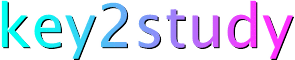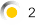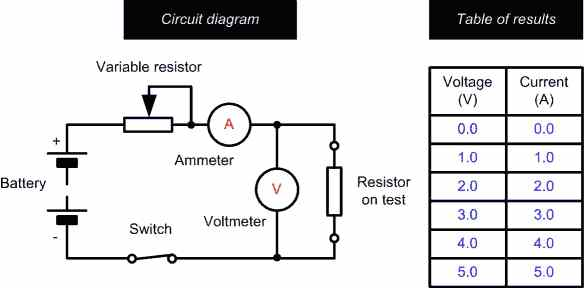Science: Ohm's Law

Resistance

For any conductor, the current flowing is directly proportional to the e.m.f. applied. The current flowing will also be dependent on the physical dimensions (length and cross-sectional area) and material of which the conductor is composed. The amount of current that will flow in a conductor when a given e.m.f. is applied is inversely proportional to its resistance. Resistance, therefore, may be thought of as an opposition to current flow; the higher the resistance the lower the current that will flow (assuming that the applied e.m.f. remains constant).

Provided that temperature does not vary, the ratio of p.d. across the ends of a conductor to the current flowing in the conductor is a constant.  This relationship is known as Ohm's law and it leads to the relationship:

V/I   =  a constant = R

where V is the potential difference (or voltage drop) in volts (V),  I is the current in amps (A), and R is the resistance in ohms.

The formula may be arranged to make V, I or R the subject, as follows:

V = I x R           I = V/R        and       R = V/I

It is important to note that, when performing calculations of currents, voltages and resistances in practical circuits it is seldom necessary to work with an accuracy of better than  1% simply because component tolerances are invariably somewhat greater than this.

An experiment to confirm Ohm's Law

We can confirm Ohm's Law by connecting a resistor to a DC supply (i.e. a battery) and varying the current applied to the resistor using a variable resistor. Corresponding values of current and voltage can be recorded in a table and a graph plotted to show the relationship between these quantities as shown below:The value of the resistor can be found by measuring the slope of the graph (recall that R = V/I). In this case the slope is 1 (i.e. 5 V / 5 A) and thus we can conclude that the resistor has a resistance of 1 ohm.

Now take a look at the results for resistors of 1 ohm, 2 ohm and 4 ohm. Note how the slope of the graph increases as the value of the resistance increases:

Some examples of using Ohm's Law

 Example A 12 ohm resistor is connected to a 6V battery.  What current will flow in the resistor? Solution Here we must use I = V/R  (where V = 6 V and R = 12 ohm):I = V/R  =  6 V / 12 ohm   =  0.5 A  (or 500 mA)Hence a current of 500 mA will flow in the resistor.
 Example A current of 100 mA flows in a 56 ohm resistor. What voltage drop (potential difference) will be developed across the resistor? Solution Here we must use V = I x R and ensure that we work in units of volts (V), amps (A), and ohms. Since 100 mA is the same as 0.1 A we can use:V = I x R = 0.1 A x 56 ohm = 5.6 VHence a p.d. of 5.6 V will be developed across the resistor.
 Example A voltage drop of 15V appears across a resistor in which a current of 1mA flows. What is the value of the resistance? Solution Now 1mA is 0.001 A thus:R = V/I = 15 V / 0.001A  = 15,000 ohm = 15k ohmNote that it is often more convenient to work in units of mA and V which will produce an answer directly in kohm. Thus:R = V/I = 15 V / 1mA = 15 kohm